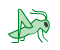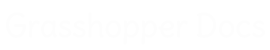﻿ Owl - Addon for Grasshopper | Grasshopper DocsCommunity documentation for Grasshopper add-ons & plugins# Owl

##### Owl is a library written for machine learning-oriented data processing.
 Backprop
 Convert
 Display
 Geometry
 I/O
 Image
 Network
 Primitive
 Reinforcement
 Scripting
 Supervised
 Tensor
 TensorSet
 Unsupervised

### TensorSet

 Add Noise (Noise)Add Noise to a TensorSet Average TSet (Average)Get TensorSet average. Bounds TSet (Bounds)Get TensorSet bounds. Interpolate TSet (Intrp)Interpolate a TensorSet. Merge TSet (MergeTS)Merge multiple TensorSets into one. Pick Tensor (Pick)Pick single Tensors out of the TensorSet. Subsample TSet (Subsample)Subsample TensorSet. Remap TSet (Remap)Remap TensorSet. Shuffle TSet (Shuffle)Shuffle TensorSet. Split TSet (SplitTS)Split TensorSet. TensorSet Stats (Stats)Get various information about the TensorSet. Trim TSet (TrimTS)Trim TensorSet values.

### Primitive

 Construct Network (Network)Construct Owl.Accord Network Load Network (Network)Load Activation Network Save Network (Network)Save Activation Network Construct Tensor (Tensor)Construct Owl Tensor Construct TensorSet (TensorSet)Construct Owl TensorSet Deconstruct Tensor (DeTensor)Deconstruct Owl Tensor Deconstruct TensorSet (DeTSet)Deconstruct Owl TensorSet Deconstruct Trigger (DeTrigger)Deconstruct Trigger

### Display

 Display Compute (DComp)Computes the network output List Devices (Devices)Image capturing devices Network Preview (2DPreview)2D network preview WebCamCapture (Capture)Capture a single frame from a webcam Preview Classes (CPreview)Quick Class preview Tensor2D Preview (2DPreview)Tensor2D preview TensorSet Display (TSView)Plot a TensorSet TensorSet Polylines (TSPoly)Plot a TensorSet as a set of polylines

### Tensor

 ReshapeReshape a Tensor. Highest Activation (High)Indicates which dimension of the Tensor has the greatest value. Tensor Addition (Add)Tensor Addition. Tensor Crop (Crop)Tensor Crop. Tensor Split (SplitT)Splits Tensor by it's leftmost dimension. Tensor Stats (Stats)Get various information about the Tensor. Tensor Subtraction (Subtract)Tensor Subtraction. Threshold (T)Apply threshold filter on the Tensor

### Unsupervised

 t-SNELaurens van der Maaten's dimensionality reduction method. t-SNE Ex (t-SNE)Laurens van der Maaten's dimensionality reduction method. Cluster Lines (ClusterL)Cluster lines KMeans Clustering (KMeans)A KMeans clustering component KMeans Clustering Ex (KMeansEx)A KMeans clustering component Markov Chain (MChain)Markov Chain series generator

### Reinforcement

 Choose Action (Action)Choose agent next action Construct QAgent (QAgent)Construct QAgent Construct QMatrix (QMatrix)Construct QMatrix Deconstruct QAgent (DeQAgent)Deconstruct QAgent Matrix2QMatrix (M2Q)Convert a spare adjacency matrix to QMatrix. UpdateQUpdate QAgent values

### Network

 Assign Values (SetNet)Assing network weights and biases directly. ComputeCompute the output values for the given input TensorSet Layer Compute (ComputeL)Compute the output values for each layer, given the input Tensor Extract Values (GetNet)Get network weights and biases as Tensors Trim Network (Trim)Trim the network

### I/O

 Load Bitmap (LoadBmp)Loads a bitmap as a Tensor. Load TensorSet (LoadTSet)Loads the TensorSet from a file. Save IDX TensorSet (ToIDX)Saves the TensorSet in an IDX file. Save TBIN TensorSet (ToTBIN)Saves the TensorSet to a binary TBIN file. Save TensorSet (ToTTXT)Saves the TensorSet to a text file.

### Convert

 DataTree to TensorSet (DT->TS)Convert a DataTree of Numbers into a TensorSet Feature TensorSet (FeatureTS)Convert multiple types of data into a TensorSet OneHot TensorSet (OneHot)Construct a OneHot TensorSet TensorSet to DataTree (TS->DT)Deconstruct Owl TensorSet into a DataTree

### Image

 Evaluate 2D (Eval2D)Evaluate 2D Tensor ResizeResize a 2D Tensor SamplesCreate sampling frames Mesh T2 (MT2)Preview 2D Tensor as a mesh

### Backprop

 BackpropagationEx (BackEx)Backpropagation, threaded. Backpropagation (BackProp)Teach the Network with backpropagation

### Supervised

 Deconstruct Network (DeNetwork)Deconstruct Owl.Learning Network Construct Network Ex (NetworkEx)Construct Owl.Learning Network from the atomic data.Use this component when importing already trained models from other frameworks.

### Scripting

 File Watch (Watcher)Watch file for changes Run Process (RunProcess)Run any application.

### Geometry

 TensorSet Cloud (TSCloud)Plot a TensorSet as a point cloud

## Video Tutorials

Site design © Robin Rodricks.   Owl and associated data © 2022 Mateusz Zwierzycki.
Rhinoceros and Grasshopper are registered trademarks of Robert McNeel & Associates.  Hosted by GitHub